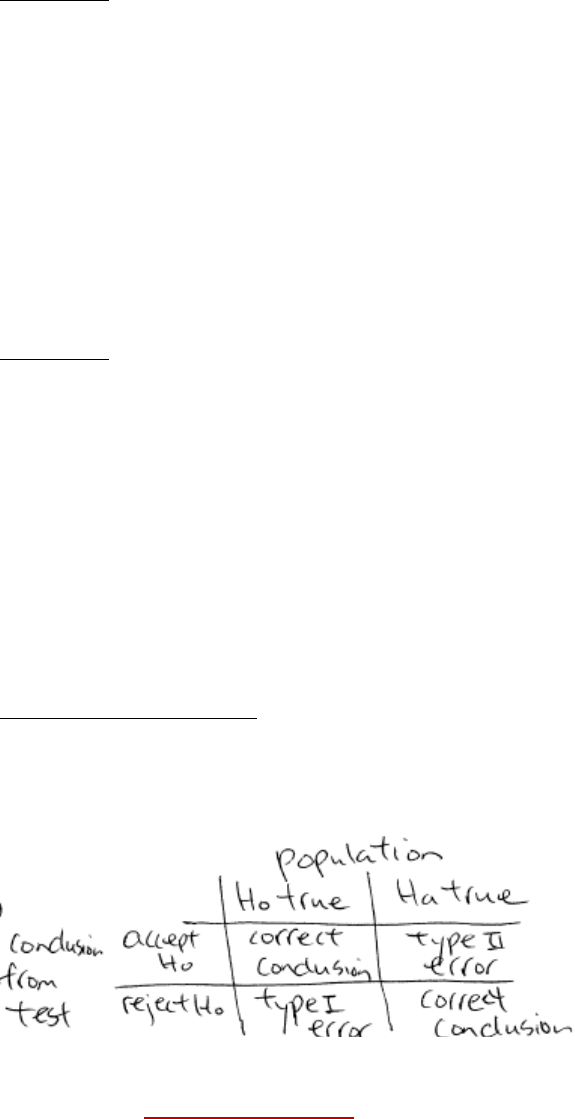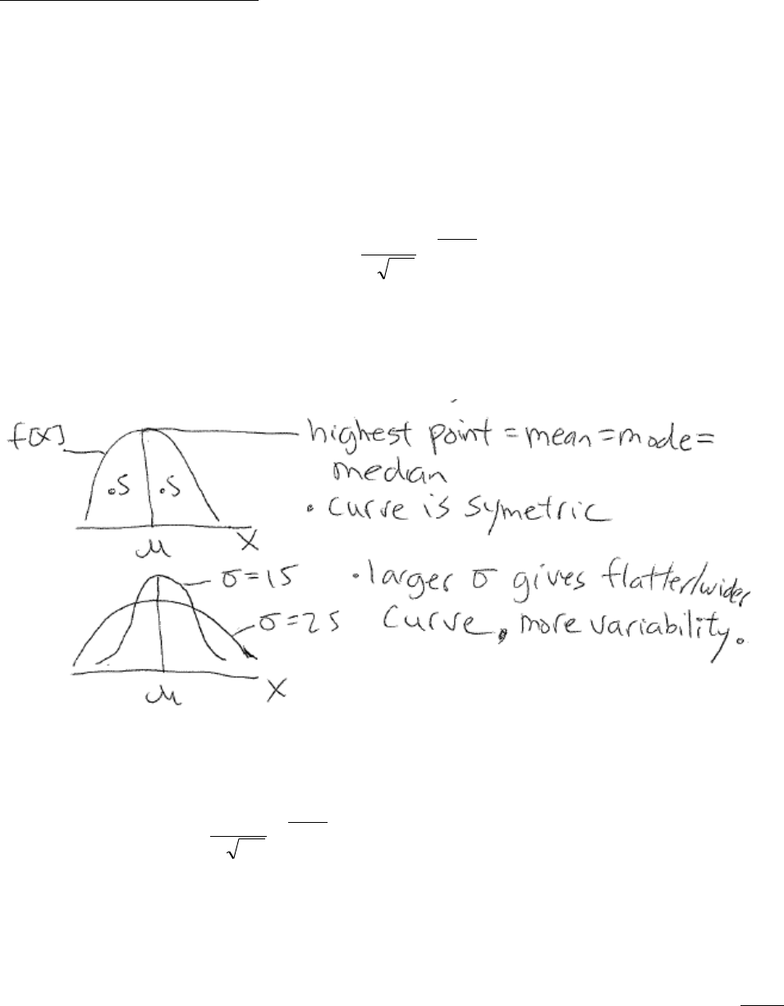Study Guides (390,000)
CA (150,000)
UTSC (10,000)
Midterm

# MGEB12H3 Study Guide - Midterm Guide: Null Hypothesis, Statistical Hypothesis Testing, Statistical Parameter

Department
Economics for Management Studies
Course Code
MGEB12H3
Professor
Victor Yu
Study Guide
Midterm

This preview shows pages 1-3. to view the full 13 pages of the document.Department of Management, UTSC
MGEB12 Quantitative Methods in Economics II - Lecture 01
Chapter 9 - Introduction to Hypothesis Testing & Testing of Mean
1. Introduction
Hypothesis testing – use to determine if statement made about value of population parameter (mean µ,
proportion p, standard deviation σ) should or should not be rejected.
Example – Ambulance company
An ambulance company says they can respond to emergencies with mean time of 12min or less. You take 40
observations and the mean time was 13.25 min. Can you conclude that the “true” mean responds time is less
than 12min? How about if mean time from observations was 12.5min?
If the true mean is ≤ 12 min what is the chance of you getting mean = 12.5min? Getting mean = 13.25?
Ho = null hypothesis, tentative assumption about population parameter
Ha = alternate hypothesis, opposite of Ho
Procedure
1. State hypothesis you want to test and the desired test significance level
2. Take observations
3. Calculate the test statistic based on the observed data
4. Determine if reject Ho or cannot reject Ho
Conventions
Manufacture claims usually given benefit of doubt and stated as null hypothesis
Research question should be expressed as Ha
The ≤ , , = should be used in Ho
The <, >,≠ should be used in Ha
1

Only pages 1-3 are available for preview. Some parts have been intentionally blurred.Example 1
Car currently can do 24 miles/gallon of gas on average. Wondering if new fuel system allows it to go further.
State hypothesis to be tested.
Example 2
Manufacture of soft drink says each bottle filled with at least 67.6 ounces. You want to know if this is true.
State hypothesis to be tested.
Type I and type II errors
Because hypothesis test base on sample data (eg. 40 observations) and not entire population, errors can
Define test significance level = α = P[making type I error] = P[incorrectly rejecting Ho given Ho true ]
Typical value of for α used in practice is .1, 0.05 or 0.01
There are 2 approaches to testing:
1. Critical value approach
2. P-value approach
2

Only pages 1-3 are available for preview. Some parts have been intentionally blurred.2. Normal Distribution Review
Many of the hypothesis test procedures assumes that population is normally distributed.
Following tends to be normally distributed
oHeight of people
oWeight of people
oScientific measurements
oTest scores
Normal probability density function:
2
2
2
)(
2
1
)(
σ
µ
πσ
=
x
exf
for -∞ ≤ x ≤ ∞
µ = mean of x
σ = standard deviation of x
The distribution of X is bell shape.
How to calculate p(x ≤ 2) = ?
dxedxxfxp
x
==
2
2
)(
2
2
2
2
1
)()2(
σ
µ
πσ
……don’t do this!
Convert random variable x into standard normal random variable Z and then look up value in Normal
Distribution Table at back of book.
Given normal random variable x, find standard Normal Random Variable Z:
σ
µ
=
x
Z
Z has mean = 0 and standard deviation = 1
3